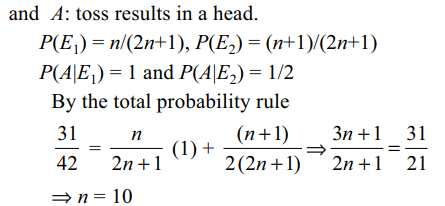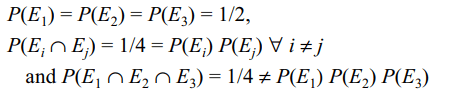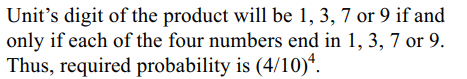## Probability Questions and Answers Part-9

1. Two non-negative integers are chosen at random. The probability that the sum of the square is divisible by 11 is
a) 9/16
b) 1/121
c) 9/17
d) 2/121

Explanation:2. A natural number x is choosen at random from the first 100 natural numbers. The probability that $x+\frac{100}{x}>50$
is
a) 1/10
b) 11/50
c) 11/20
d) 3/20

Explanation:3. A bag contains (2n + 1) coins. It is known that n of these coins have a head on both sides, whereas the remaining (n + 1) coins are fair. A coin is picked up at random from the bag and tossed. If the probability that the toss results in a head is 31/42, then n is equal to
a) 10
b) 11
c) 12
d) 13

Explanation:4. If X and Y are independent binomial variate B (5, 1/2) and B (7, 1/2), then P (X + Y = 3) is
a) 55/1024
b) 55/4098
c) 55/2048
d) 55/512

Explanation:5. Suppose $n\left(\geq 3\right)$   persons are sitting in a row. Two of them are selected at random. The probability that they are not together is
a) $1-\frac{2}{n}$
b) $\frac{2}{n-1}$
c) $1-\frac{1}{n}$
d) $\frac{2}{n}$

Explanation:6. A bag contains four tickets marked with numbers 112, 121, 211, 222. One ticket is drawn at random from the bag. Let $E_{i}\left(i=1,2,3\right)$   denote the event that ith digit on the ticket is 2. Then which of the following is not true
a) $E_{1}$ and $E_{2}$ are independent
b) $E_{2}$ and $E_{3}$are independent
c) $E_{3}$ and $E_{1}$ are independent
d) $E_{1},E_{2}, E_{3}$   are independent

Explanation:7. If the letters of the word PROBABILITY are written down at random in a row, the probability that two B's are together is
a) 2/11
b) 10/11
c) 3/11
d) 6/11

Explanation:8. If four positive integers are taken at random and multiplied together, then the probability that the last digit is 1, 3, 7 or 9 is
a) 1/8
b) 2/7
c) 1/625
d) 16/625

Explanation:9. Two contestants play a game as follows: each is asked to select a digit from 1 to 9. If the two digits match they both win a prize. The probability that they will win a prize in a single trial is
a) 1/8
b) 7/81
c) 1/9
d) 3/11

b) $^{2n}C_{n}\left(\frac{1}{2}\right)^{2n}$
c) $1-2^{n}C_{n}\left(\frac{1}{2}\right)^{2n}$
d) $\frac{1}{2}-^{2n}C_{n}\left(\frac{1}{2}\right)^{2n+1}$